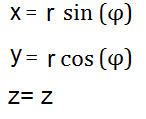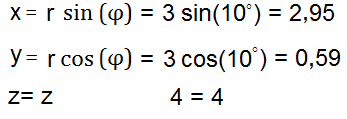﻿ Cylindrical to Cartesian Coordinates Calculator ﻿# Cylindrical to Cartesian Coordinates Calculator### Cylindrical to Cartesian (Rectangular) Coordinate System DiagramThis cartesian (rectangular) coordinates converter/calculator converts the cylindrical coordinates of a unit to its equivalent value in cartesian coordinates, according to the formulas shown above.

Cylindrical coordinates are depicted by 3 values, (r, φ, Z). When converted into cartesian coordinates, the new values will be depicted as (X, Y, Z).

To use this calculator, a user just enters in the (r, φ, z) values of the cylindrical coordinates and then clicks 'Calculate', and the cartesian coordinates will be automatically computed and shown below.

This calculator can be used to convert 2-dimensional (2D) or 3-dimensional cylindrical coordinates to its equivalent cartesian coordinates. If desired to convert a 2D cylindrical coordinate, then the user just enters values into the r and φ form fields and leaves the 3rd field, the z field, blank. Z will will then have a value of 0. If desired to convert a 3D cartesian coordinate, then the user enters values into all 3 form fields, r, φ, z.

By default, the calculator will compute the result in degrees. However, by using the drop-down menu, the option can changed to radians, so that the result can also be computed in radians.

Example Calculation

Convert the cylindrical coordinates (3, 10°, 4) into its equivalent cartesian coordinate.This answer is calculated in degrees mode.

Related Resources

﻿